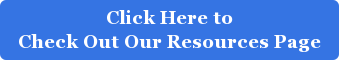#### ACT Trigonometry Tips

In past blogs we have highlighted the major differences between the SAT and ACT. While the ACT and SAT math sections do share a lot of topics in common, the ACT also tests for the following topics, which are not covered by the SAT:  Trigonometry, Logarithms, Complex Numbers, and Matrices/Circles & Ellipse Equations.

Depending on whether you enjoy your high school math classes, this may or may not be good news. Whether you are a math genius, or require a calculator for even the most basic math equation, the tips below should help solidify some of the fundamentals of trigonometry.

#### Understand What Trigonometry Is

According to Merriam Webster’s, trigonometry is a “mathematical discipline dealing with the relationships between the sides and angles of triangles. Literally, it means triangle measurement, though its applications extend far beyond geometry”

#### Understand Common Trigonometric Terms

When you encounter a trigonometry question, you will typically see the following terms:

Hypotenuse: The longest side of a triangle

Sine: The sine of any given angle is the ratio of the length of its opposite side to the length of its hypotenuse

Cosine: The cosine of any given angle is the ratio of the length of its adjacent side to the length of the hypotenuse

Tangent: The tangent of any given angle is the ratio of the length of its opposite side to the length of its adjacent side.

Cosecant: The reciprocal of the sine of a given angle

Secant: The reciprocal of the cosine of a given angle

Cotangent: The reciprocal of the tangent of a given angle

SOH-CAH-TOA is a popular acronym for remembering the relationship between triangles sides and angles.In trigonometry there are certain types of triangles (with specific ratios of triangle sides) that appear frequently on the ACT. Memorizing these will allow you to quickly identify and solve the questions. When given only two sides, recognizing this ratio will allow you to accurately calculate the length of the missing side. These popular triangles have sides in the following ratios: 3-4-5 & 5-12-13

Other popular triangles are the 45-45-90 triangle and the 30-60-90 triangle. Familiarity with these triangles and angle ratios will ensure that you are able to figure out the accompanying side ratios. See the image below to understand the correlation:

45-45-90 Triangle30-60-90 Triangle#### Have more ACT Trigonometry questions? Just Ask Suniti!

Image sources: mathwarehouse.com, Wikimedia, unlimited gmat

Tags: For StudentsSuniti is the creator of TestRocker, an online learning platform that helps you unlock your dream PSAT, SAT and ACT scores. TestRocker is based on Suniti’s highly successful and proven method of teaching thousands of students how to maximize their PSAT, SAT and ACT scores for over a decade.

TestRocker is an award-winning PSAT/SAT/ACT program that empowers students to take control of their test preparation. After taking a diagnostic test, students receive a customized study plan, individualized to their strengths/weaknesses. Students practice and learn concepts through videos. Each of the 2000+ questions on TestRocker is accompanied by a video explanation from Suniti. TestRocker has the largest video library in the world for PSAT/SAT/ACT preparation.